Question

# A crate of mass m = 1.12 kg is on a frictionless incline that makes an...

A crate of mass m = 1.12 kg is on a frictionless incline that makes an angle of θ = 27.6 degrees with horizontal. A force of magnitude P = 7.24 N is applied to the crate in a direction parallel and up the incline, as shown.

Write an expression for the acceleration ax of the crate, where positive x is up the incline. Your answer should be in terms of m, P, θ, and g (9.80 m/s2).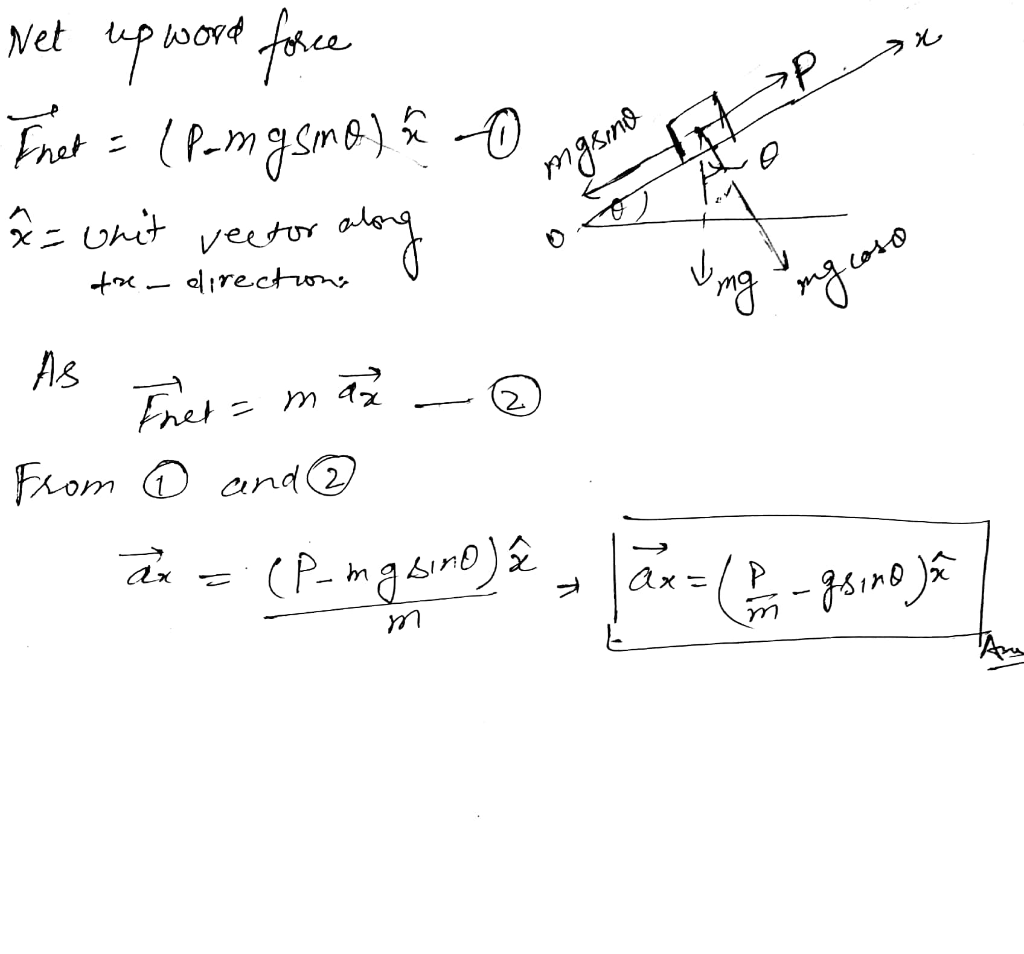#### Earn Coins

Coins can be redeemed for fabulous gifts.

Similar Homework Help Questions
• ### A horizontal force of 99 N pushes a 11 kg block up a frictionless incline that...

A horizontal force of 99 N pushes a 11 kg block up a frictionless incline that makes an angle of 41° with the horizontal. (a) What is the normal force that the incline exerts on the block?                    =N (b) What is the acceleration of the block? (Hint: assume up the block as the positive direction.)                  =m/s2

• ### To push a 26.0 kg crate up a frictionless incline, angled at 25.00 to the horizontal,...To push a 26.0 kg crate up a frictionless incline, angled at 25.00 to the horizontal, a worker exerts a force of 214 N, parallel to the incline. Assume that the crate slides 1.50 m. (a) What is the work done on the crate by the worker's applied force? (b) What is the work done by the gravitational force on the crate? (c) What is the work done by the normal force exerted by the incline on the crate? (d)...

• ### A charged box (m - 455 g, q +2.50 uC) is placed on a frictionless incline...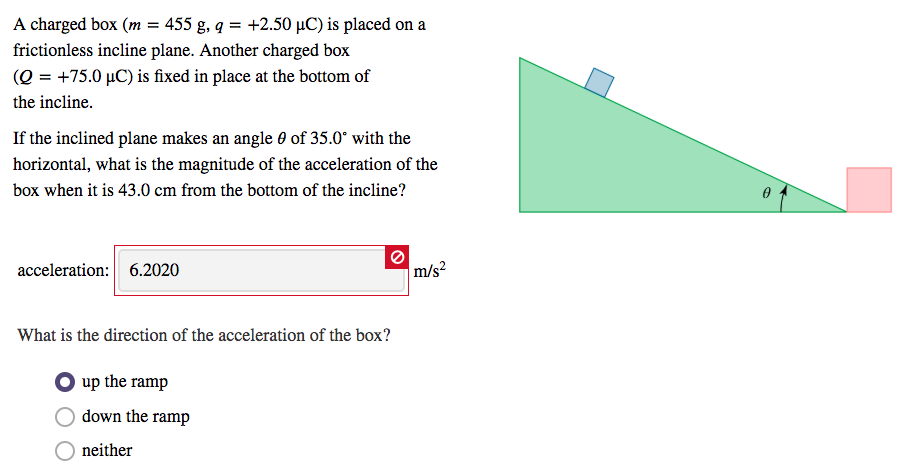A charged box (m - 455 g, q +2.50 uC) is placed on a frictionless incline plane. Another charged box (Q-+75.0 μC) is fixed in place at the bottom of the incline. If the inclined plane makes an angle θ of 35.0° with the horizontal, what is the magnitude of the acceleration of the box when it is 43.0 cm from the bottom of the incline? acceleration: 6.2020 What is the direction of the acceleration of the box? O up...

• ### A 9.2 kg crate is pulled up a rough incline with an initial speed of 1.2...

A 9.2 kg crate is pulled up a rough incline with an initial speed of 1.2 m/s A pulling force of 105N is applied parallel to the surface of the incline, which is at an angle of 21.8 degrees to the horizontal. The coefficient of kinetic friction is .32 and the crate is pulled 7.3 m Find the change in KE of the crate find the speed of the crate after it is pulled 7.3 m

• ### Figure 1: Block on incline. 2, A block of mass m = 5 kg is subject...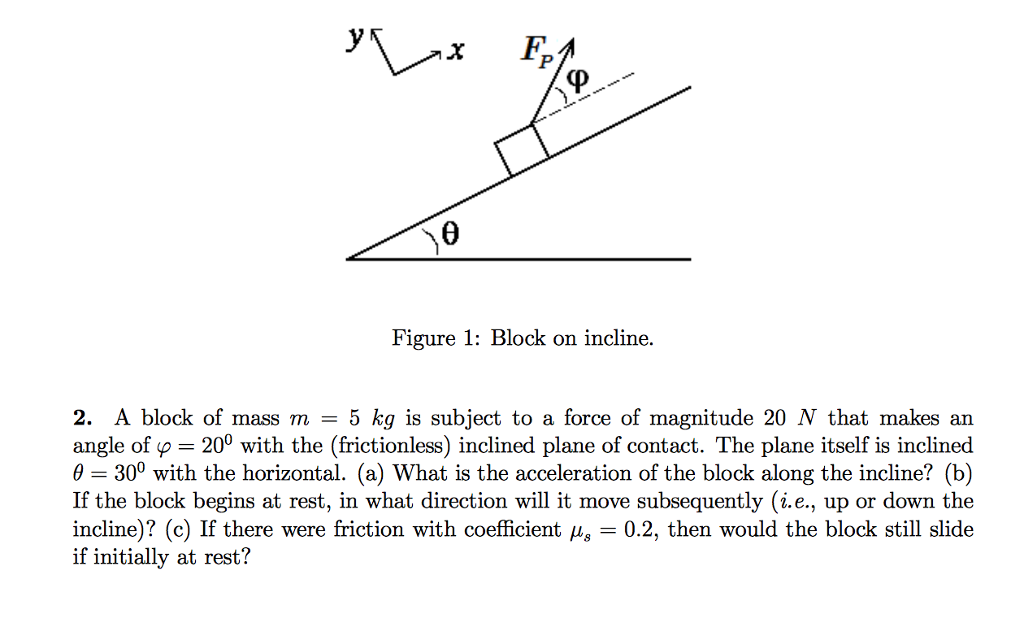Figure 1: Block on incline. 2, A block of mass m = 5 kg is subject to a force of magnitude 20 N that makes an angle of p20 with the (frictionless) inclined plane of contact. The plane itself is inclined θ = 300 with the horizontal. (a) What is the acceleration of the block along the incline? (b) If the block begins at rest, in what direction will it move subsequently (i.e., up or down the incline)? (c) If...

• ### A crate of mass 10 kg is pulled up a rough incline with an initial speed...

A crate of mass 10 kg is pulled up a rough incline with an initial speed of 1.5 m/s. The pulling force is 100 N, parallel to the incline; which makes an angle of 20o with the horizontal. The coefficient of friction is 0.40, and the crate is pulled 5.0 m. Has the mechanical energy of the system increased or decreased? Show your work.

• ### A crate of mass 9.6 kg is pulled up a rough incline with an initial speed...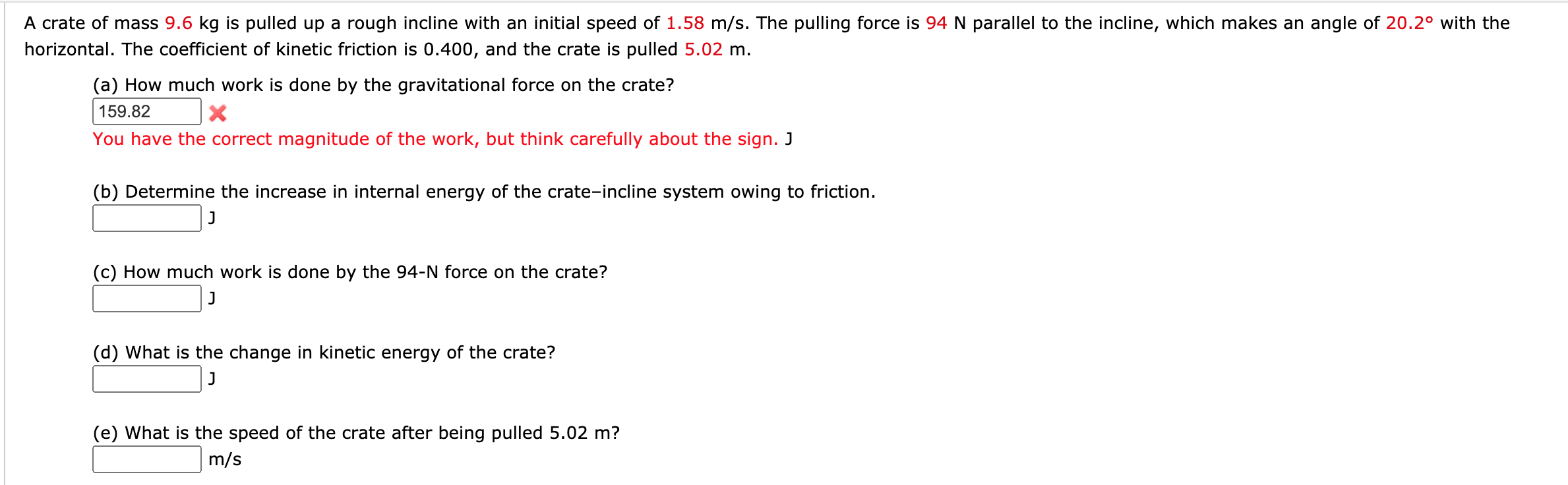A crate of mass 9.6 kg is pulled up a rough incline with an initial speed of 1.58 m/s. The pulling force is 94 N parallel to the incline, which makes an angle of 20.2° with the horizontal. The coefficient of kinetic friction is 0.400, and the crate is pulled 5.02 m. (a) How much work is done by the gravitational force on the crate? 159.82 You have the correct magnitude of the work, but think carefully about the sign....

• ### A small block of mass m AMT and charge Q is placed on an insulated, frictionless,...

A small block of mass m AMT and charge Q is placed on an insulated, frictionless, inclined plane of angle θ as in Figure below. An electric field is applied parallel to the incline.    a) Find an expression for the magnitude of the electric field that enables the block to remain at rest. b) If m = 5.40 g, Q = -7.00 µC, and θ = 25.0o, determine the magnitude and the direction of the electric field that enables the...

• ### 3. A crate of mass 10 kg is pulled up a rough incline which makes an...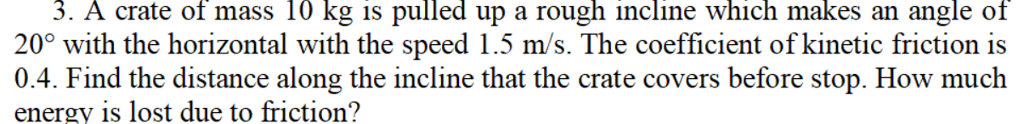3. A crate of mass 10 kg is pulled up a rough incline which makes an angle of 20° with the horizontal with the speed 1.5 m/s. The coefficient of kinetic friction is 0.4. Find the distance along the incline that the crate covers before stop. How much energy is lost due to friction?

• ### A crate of mass 11.0 kg is pulled up a rough incline with an initial speed...

A crate of mass 11.0 kg is pulled up a rough incline with an initial speed of 1.40 m/s. The pulling force is 90.0 N parallel to the incline, which makes an angle of 19.6° with the horizontal. The coefficient of kinetic friction is 0.400, and the crate is pulled 4.90 m. (a) How much work is done by the gravitational force on the crate? (b) Determine the increase in internal energy of the crate-incline system due to friction. (c)...